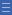# Activity: Strike it out

Activity: Strike it out

A game that involves calculations from crossing out numbers on a number line from 0 to 20.

## Find the activity on NRICH

Go to http://nrich.maths.org/6589 (link will open in a new window)

## Variations

Easier: Use a number line from 0 to 10

Harder: Use multiplication and division as well as addition and subtraction

## More questions

Can you cross out all the numbers in one game? How do you know?

What is the largest amount of numbers you can cross out?

What digits should you place in each square to make the largest sum possible? Is there more than one way to do this? What about the smallest sum?

Are all totals between the smallest and the largest ones possible? If not, which ones aren’t?

If you place the digits 1 to 9 in a 3 x 3 square what is the smallest possible sum of the six 3-digit numbers formed by the rows and columns? Can you make the sum 2000?

## Shine a light on the Proficiencies

Games of strategy are a good way to develop skills in logical thinking, and problem solving skills such as working backwards.

Explaining that for a number line starting with 0 it is never possible to cross out 0 itself provides a good opportunity for even very young students to use mathematical reasoning.

Students could look at how many numbers are crossed out and/or circled after each move, and reason that if we want to cross out every number except 0, the number line must end in an odd number.

Yes

Yes

Name Class Section PriorityDownload Strike It Out (blank worksheet) File 1